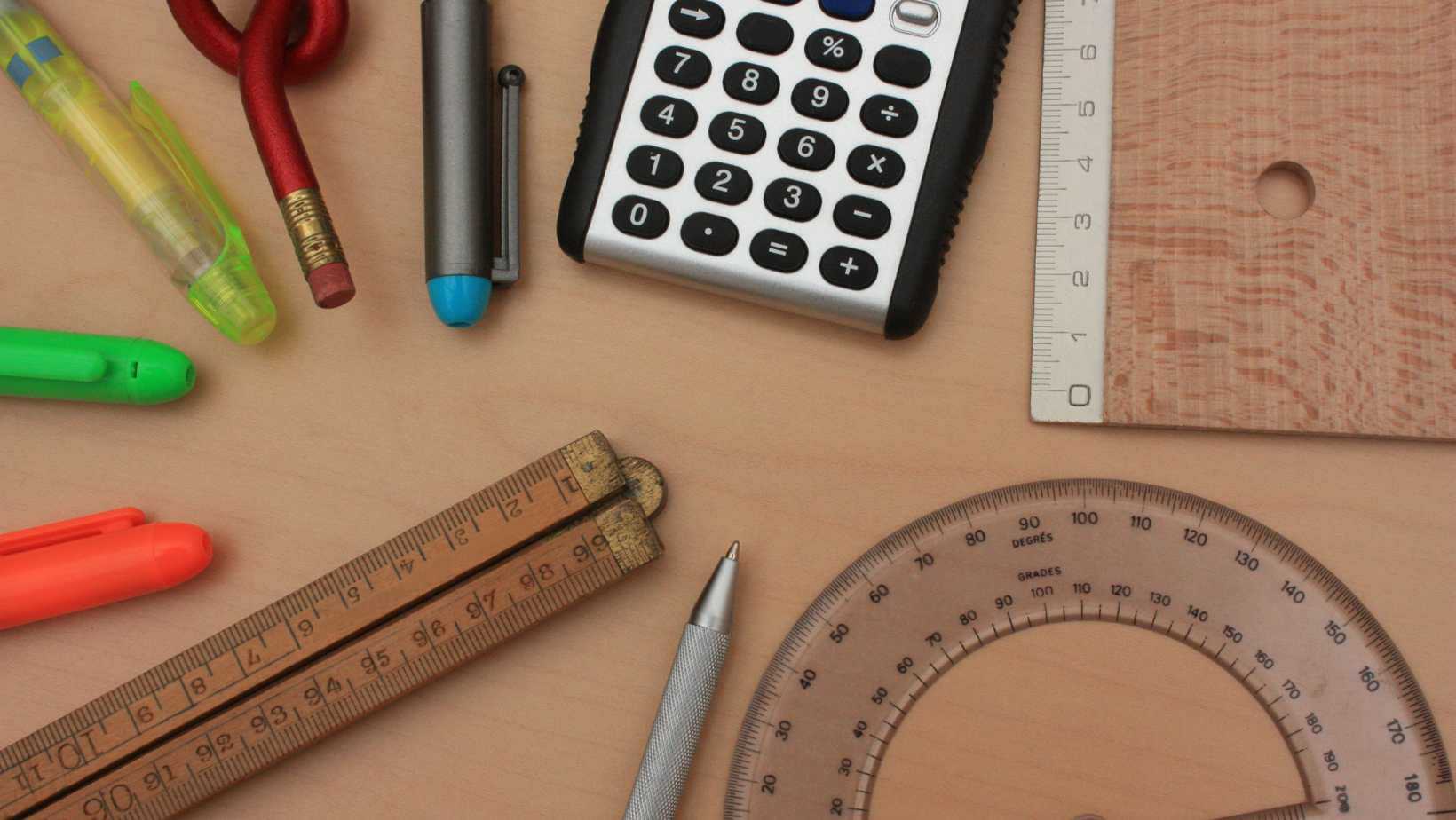# How Many ML in a QT: A Simple Conversion GuideIf you’ve ever found yourself in the kitchen, following a recipe and scratching your head over measurements, you’re not alone. One common question that arises is, “How many ml are in a qt?” Well, let me break it down for you. In the United States customary system of measurement, there are approximately 946.35 milliliters in one quart.

To put it simply, a quart (qt) is a unit of volume commonly used in cooking and baking. On the other hand, milliliters (ml) are often used to measure smaller quantities of liquid. So if you need to convert quarts to milliliters or vice versa, knowing that one quart is equivalent to around 946.35 milliliters can be quite handy.

## How Many ML In A QT

When it comes to understanding the basic measurement of how many milliliters are in a quart, it’s important to have a clear grasp of the conversion rate. A quart is commonly used for measuring liquids, especially in cooking and baking recipes. On the other hand, milliliters are often used in scientific measurements and pharmaceutical dosages.

To convert milliliters to quarts, you need to know that there are 946.353 milliliters in one US liquid quart. This conversion factor allows you to easily switch between these units of measurement. For example:

• If you have 500 milliliters of water and want to know how many quarts that is, divide 500 by 946.353 (500/946.353). The result is approximately 0.52834 quarts.
• Conversely, if you have 2 quarts of milk and want to convert it into milliliters, multiply 2 by 946.353 (2 * 946.353). The result is approximately 1892.706 milliliters.

Remembering this simple conversion rate can be helpful when working with different recipes or when dealing with specific scientific measurements.

### Common Mistakes When Converting Milliliters to Quarts

While converting milliliters to quarts may seem straightforward, there are a few common mistakes that people make. It’s important to be aware of them to avoid any errors in your measurements:

1. Rounding Errors: When using the conversion factor of 946.353 milliliters per quart, it’s crucial to use the full decimal value for precise conversions. Rounding off too early can lead to inaccuracies in your calculations.
2. Forgetting Decimals: Sometimes, people forget to include the decimal point when converting between quarts and milliliters. This mistake can result in significantly larger or smaller quantities than intended.
3. Confusing Units: Mixing up different units of measurement can also lead to errors. Make sure you’re clear on whether you’re working with quarts or fluid ounces before attempting any conversions.

By being aware of these common pitfalls, you can ensure that your measurements remain accurate and reliable when converting between milliliters and quarts.

## Converting From Milliliters To Quarts

When it comes to converting measurements, understanding the relationship between milliliters (ml) and quarts (qt) can be quite useful. Knowing how many milliliters are in a quart will help you accurately convert volumes between these two units. So, let’s dive into the conversion process and explore how many ml are in a qt.

To start off, it’s important to note that both milliliters and quarts are units of volume measurement. Milliliters are commonly used for smaller quantities, while quarts are often used for larger volumes. Now, let’s get down to the specifics:

1 quart is equivalent to 946.353 milliliters.

This conversion factor allows us to convert any given volume from milliliters to quarts or vice versa. By multiplying the number of quarts by 946.353, we can obtain the corresponding volume in milliliters.

For example:

• If we have 2 quarts, we can calculate the equivalent volume in milliliters by multiplying 2 by 946.353.
• Result: 2 quarts = 1892.706 ml

On the other hand, if we want to convert from milliliters to quarts, we need to divide the given volume in milliliters by 946.353.

For example:

• If we have 2000 ml and want to find out how many quarts this is equal to, we divide 2000 by 946.353.
• Result: 2000 ml ≈ 2.1134 qt (approximately)

It’s worth noting that when dealing with conversions between different units of measurement like this one, precision may vary slightly due to rounding errors.

In conclusion,

• To convert from milliliters (ml) to quarts (qt), divide the given volume in milliliters by approximately 946.353.
• To convert from quarts (qt) to milliliters (ml), multiply the given volume in quarts by approximately 946.353.

Having a good grasp of these conversion factors will enable you to effortlessly transform volumes between milliliters and quarts, making your cooking, baking, or scientific experiments a breeze!

Amanda is the proud owner and head cook of her very own restaurant. She loves nothing more than experimenting with new recipes in the kitchen, and her food is always a big hit with customers. Amanda takes great pride in her work, and she always puts her heart into everything she does. She's a hard-working woman who has made it on her own, and she's an inspiration to all who know her.